Latest Banking jobs   »

# Quantitative Aptitude Quiz For RBI Grade B/ ECGC PO/ SIDBI Grade A Prelims 2022- 27th May

A survey conducted on 6400 people in a town about which mobile network gives high speed data. 25% of the total town population selected only Airtel network. 15% of the total town population selected only Vodafone network. 7% of the total town population selected only Idea network. 12% of the total population of town selected only Aircel network. 16% of the total town population selected only Jio network. 6% of the total population of town selected Airtel & Vodafone only. 8% of the total town population selected Airtel, Vodafone & Jio only. 5% of the total population of town selected Vodafone, Aircel & Jio only and 6% of the total population of town selected all the five networks.

Q1. Total population of town who selected only Idea, only Aircel &only Jio network together are what percent less than total population of town who selected only Airtel & only Vodafone together?
(a) 10.5%
(b) 12.5 %
(c) 11.5%
(d) 15.5%
(e) 16.5%

Q2. Find total population of town who selected at most two mobile networks?
(a) 5164
(b) 5162
(c) 5184
(d) 5158
(e) 5188

Q3. Find total population of town who selected at least two mobile networks?
(a) 1200
(b)1400
(c)1800
(d)1600
(e)2000

Q4. Find ratio between total population of town who selected all Vodafone, Aircel & Jio only to total population of town selected all the five networks?
(a) 6 : 5
(b) 5 : 4
(c) 5 : 7
(d) 5 : 9
(e) 5 : 6

Q5. Total population who selected Jio network only is what percent more than total population who selected all Airtel, Vodafone & Jio only?
(a)120%
(b) 140%
(c) 160%
(d) 100%
(e) 96%

Directions (6-10): Given below the table shows Investment of five person, time and share of profit. Some data are missing, calculate that according to the question and answer the questions –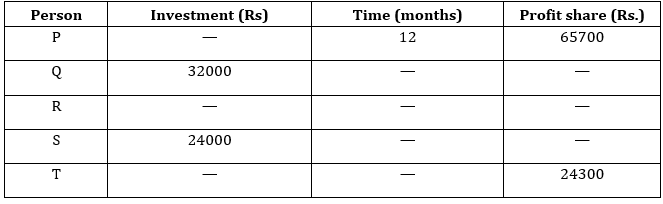Q6. P, Q and S invested in a business together, investment of P is 75% of investment of S and all three invested for same time. If Q and S gets extra 12% and 15% of total profit respectively and remaining profit is distributed according to their share, then find the total profit.
(a) 270000 Rs.
(b) 370000 Rs.
(c) 375000 Rs.
(d) 275000 Rs.
(e) 325000 Rs.

Q7. Person Q and R invested in the ratio of 4 : 3 and ratio between time period of Investment Q to R is 5 : 3. If both person agree that 65% of the total profit should be divided equally and remaining profit is to be divided into ratio of their capital. If Q gets 10010 Rs. more to R. then find the total profit share of R ?
(a) 27765 Rs.
(b) 28190 Rs.
(c) 42497 Rs.
(d) 34307 Rs.
(e) 32695 Rs.

Q8. Q started a business with his investment, after some month S came to joined with him and invest his amount in business. At the end of 3 year, the ratio of profit of Q to S is 3 : 2. Find after how many month S joined the business ?
(a) 4 months
(b) 6 months
(c) 3 months
(d) 5 months
(e) 6 months

Q9. If T received Rs. 24300 as profit out of the total profit of Rs. 40500 which T and R earned at the end of one year. If T invested Rs. 81000 for 9 months, whereas R invested his amount for the whole year, what was the amount invested by R ?
(a) 30500 Rs.
(b) 40500 Rs.
(c) 35500 Rs.
(d) 32500 Rs.
(e) 40050 Rs.

Q10. If Q and R invested into the ratio of 8 : 9 and R and S Invested into the ratio of 3 : 2. At the end of the year if they all got a total profit of 37030 Rs. then find the share of profit of Q, R and S individually ?
(a) 14490 Rs, 9660 Rs, 12880 Rs.
(b) 9660 Rs, 17710 Rs, 11005 Rs.
(c) 12880 Rs, 14490 Rs, 9660 Rs.
(d) 12434 Rs, 13594 Rs, 11006 Rs.
(e) None of these

Q11. Rahul took a loan of Rs.80000/- at rate of of 5% p.a. at SI & lent it at 5% pa at CI. After 3 years, he cleared all his debts and invested the profit earned in a scheme which offers C.I. After two years he got Rs.128.1/- as interest from the scheme. Find the rate of interest offered by scheme.
(a) 8%
(b) 7%
(c) 6%
(d) 10%
(e) 12%

Q12. Marked price of two articles A and B are in the ratio of 7 : 9, at the time of selling shopkeeper gives discount of d% on article A and (d + 5)% on article B and made a profit of 25% on each article, if cost price of article A and B are in ratio 112 : 135. Then find percent of discount given by shopkeeper on both articles?
(a) 12.5%, 17.5%
(b) 25%, 30%
(c) 10%, 15%
(d) 15 %, 20%
(e) 20%, 25%

Q13. 20% increase in both radius and height of the cylinder increases the total surface area of cylinder by 677.6 cm², If the ratio of radius to height is 1 : 4, then find the radius of cylinder
(a) 21 cm
(b) 10.5 cm
(c) 3.5 cm
(d) 14 cm
(e) 7 cm

Q14. Two pipes P and Q can fill tank A in 28 minutes and 56 minutes respectively and empty pipe M can empty same tank in 42 minutes. If all three pipes opened in tank B for (x – 24) minutes together they filled 90 liter of the tank which is 25% of the quantity tank B. Find in ‘x’ minutes what portion of tank B filled, if all pipe P and Q and M opened alternatively starting with P, followed by Q and M respectively?
(a) 7/12
(b) 7/36
(c) 5/36
(d) 3/35
(e) 7/41

Q15. There are three vessels A, B & C having Milk to water ratio as 3 : 5, 1 : 3 and 7 : y. After adding mixture of vessel B to A, the milk to water ratio becomes 1 : 2 in A . Now 60% of mixture is taken out from vessel A and mixture of vessel C is mixed with remaining mixture of vessel A which results in final Milk to Water ratio of 3 : 4. Find the value of y given that volume of B is 20 ltrs and volume of C is (7 + y) ltrs.
(a) 1
(b) 4
(c) 5
(d) 3
(e) 2

Solutions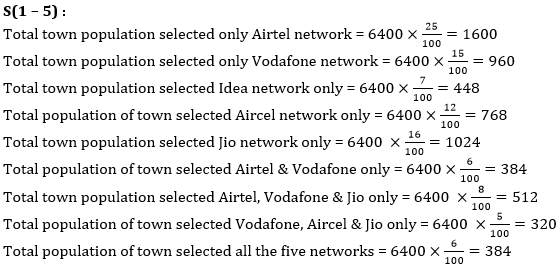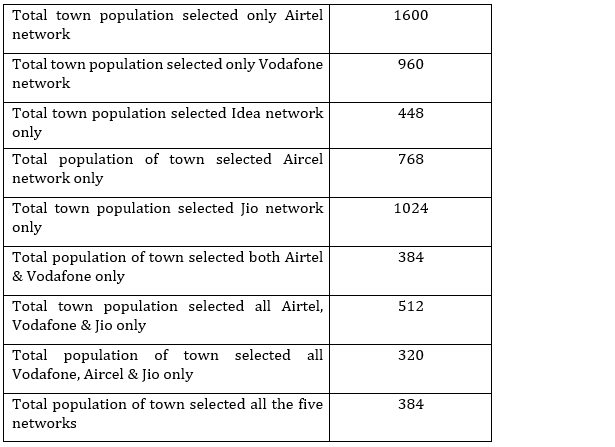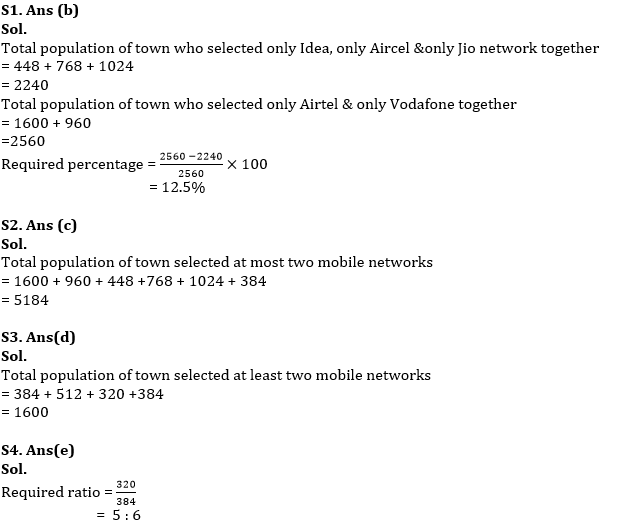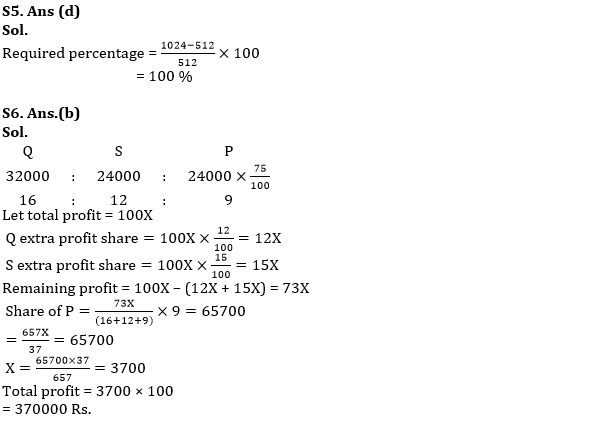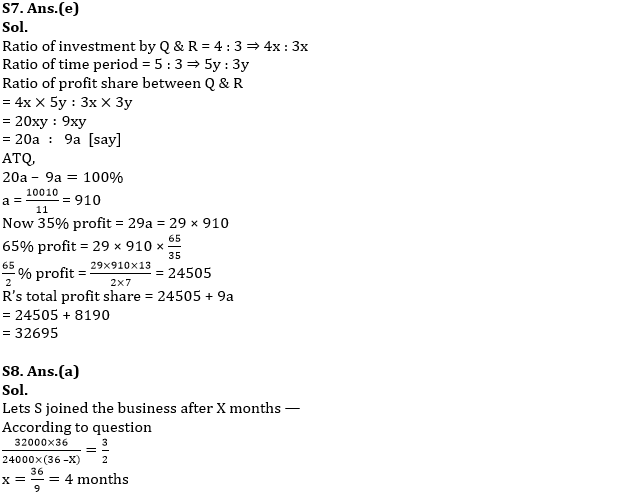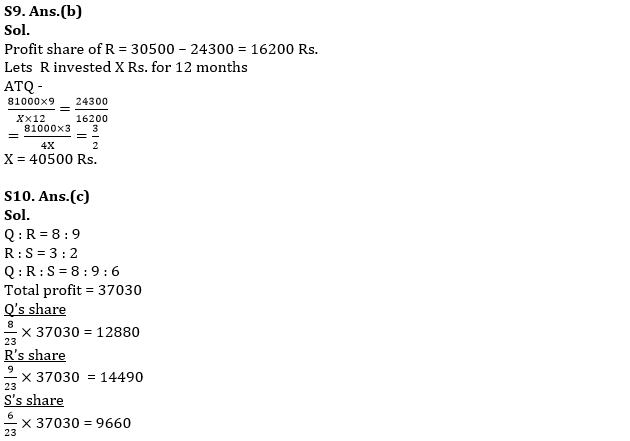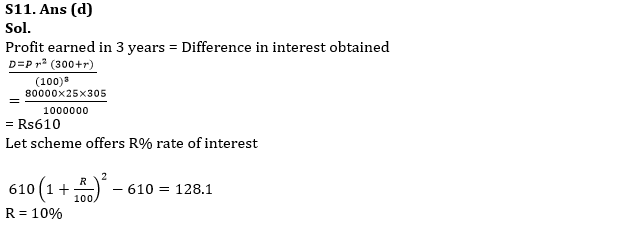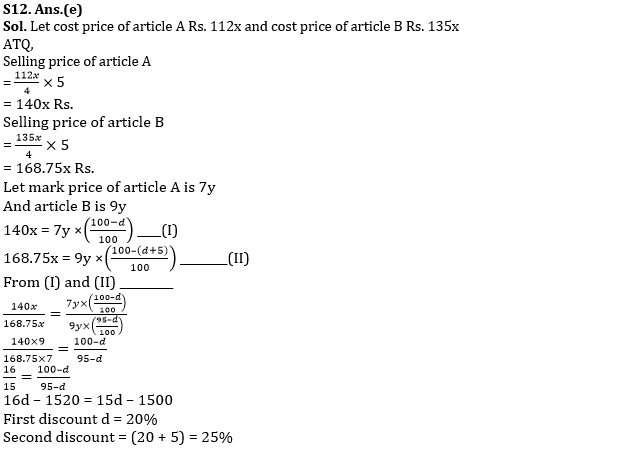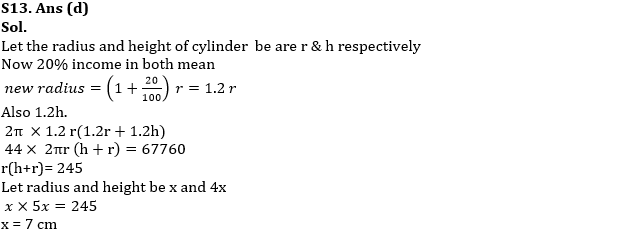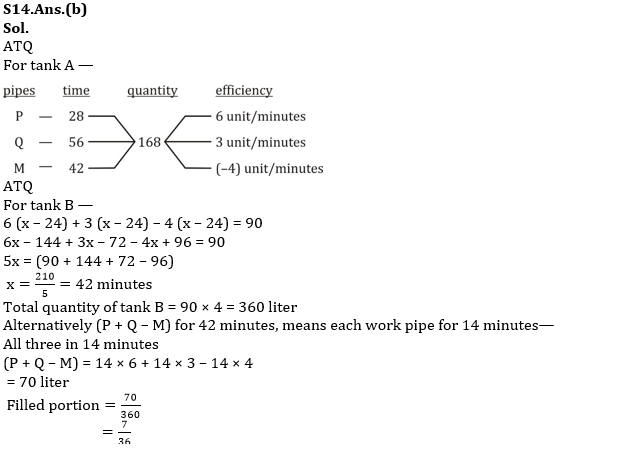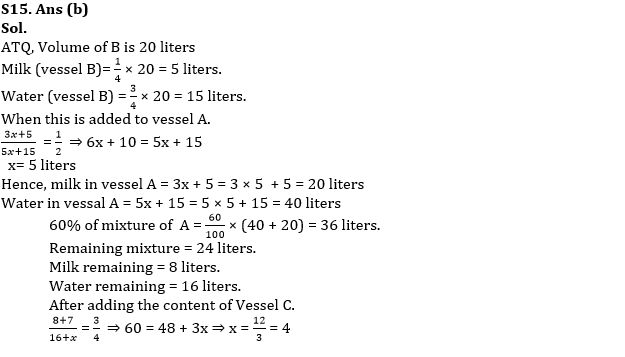#### Congratulations!